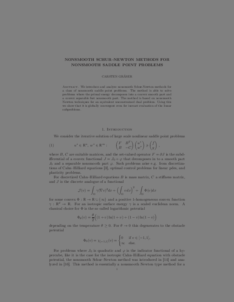Repository: Freie Universität Berlin, Math Department

# Nonsmooth Schur-Newton methods for nonsmooth saddle point problems.

Gräser, C. (2013) Nonsmooth Schur-Newton methods for nonsmooth saddle point problems. Preprint 1004, Matheon Berlin .Preview

341kB

## Abstract

We introduce and analyze nonsmooth Schur-Newton methods for a class of nonsmooth saddle point problems. The method is able to solve problems where the primal energy decomposes into a convex smooth part and a convex separable but nonsmooth part. The method is based on nonsmooth Newton techniques for an equivalent unconstrained dual problem. Using this we show that it is globally convergent even for inexact evaluation of the linear subproblems.

Item Type: Article Mathematical and Computer Sciences > Mathematics > Numerical Analysis Department of Mathematics and Computer Science > Institute of Mathematics 1824 Ekaterina Engel 06 Mar 2016 12:30 03 Mar 2017 14:42

Repository Staff Only: item control page